### CBSE Class 7 Maths Sample Paper 2# CBSE CLASS 7 MATHS SAMPLE PAPER – 2

CBSE Board

Class VII Mathematics

Sample Paper -2

Time: hrs                                                                                                                    Total Marks: 80

General Instructions:

• All questions are compulsory.
• Section A comprises of 12 questions carrying 1 mark each.
• Section B comprises of 12 questions carrying 2 marks each.
• Section C comprises of 8 questions carrying 3 marks each.
• Section D comprises of 5 questions carrying 4 marks each.

Section A

(Questions 1 to 12 carry 1 mark each)

1.

$-5+9+(-5)+(-10)+(1)$ is equal to

A. 13

B. $-13$

C. $-10$

D. 10

2.

$0.2 times 0.2=$

A. $0.4$

B. $0.04$

C. $0.004$

D. $4.0$

3.

The mode of following data is

$14,11,12,13,11,12,11$

A. 14

B. 11

C. 12

D. 13

4.

The linear equation $frac{mathrm{r}}{3}=mathrm{q}$ can be written in statement form as

A. 3 times $mathrm{p}$ is $mathrm{q}$

B. One third of $mathrm{p}$ is $mathrm{q}$

C. q times $mathrm{p}$ is 3

D. One ninth of $mathrm{q}$ is $mathrm{p}$

5.

Number of acute angles in the following figure is

A. 2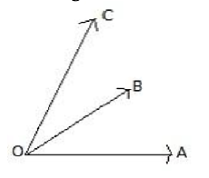B. 1

C. 4

D. 3

6.

In the adjoining figure, if $mathrm{AB}=mathrm{PQ}$ and $mathrm{BC}=mathrm{CQ}$, then find the measure of angle $mathrm{CPQ}$.

A. $80^{circ}$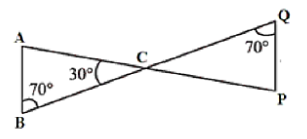B. $90^{circ}$

C. $30^{circ}$

D. $60^{circ}$

7.

$25 times(-99)$ can be written as

A. $25 times(-100)+25 times 1$

B. $25 times(100)+25 times 1$

C. $25 times(100)-25 times 1$

D. $25 times(-100)-25 times 1$

8.

If $12.543$ is distributed in 100 parts, then each part equals

A. $125.43$

B. $1254.3$

C. $1.2543$

D. $0.12543$

9.

If on adding 9 to twice of a whole number gives 31 , then the whole number is

A. 21

B. 16

C. 11

D. 7

10.

What is the difference between the measures of a straight angle and a right angle?

A. $45^{circ}$

B. $60^{circ}$

C. $100^{circ}$

D. $90^{circ}$

11.

In the figure given below, name the parts of the triangles that can be used to prove the

congruence of the two triangles.

A. $A B=A D ; B C=D E ; angle B=angle D$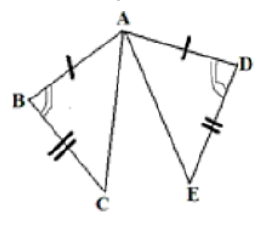B. $A C=A E ; A B=A D ; B C=D E$

C. $angle B=angle D ; angle C=angle E$

D. $angle mathrm{B}=angle mathrm{D} ; angle mathrm{C}=angle mathrm{E} ; mathrm{AB}=mathrm{AD}$

12.

The first step that we will use to separate variables and constants in the linear equation $2 mathrm{x}+$$3=7$ is

A. Transposing 3 to RHS

B. Transposing 7 to LHS

C. Diving both sides by 2

D. Multiplying both sides with 3

Section B

(Questions 13 to 24 carry 2 marks each)

13.

A bag has 12 balls colored yellow, blue, green and red. The number of balls of each colour is

the same. A ball is drawn from the bag. Calculate the probability of drawing a yellow ball, a

blue ball, a green ball and a red ball.

14.

Write the linear equation for the following statement:

“5 added to three-fifth of number gives $frac{14}{3}$

Hence, find the number.

15.

If $a=-8, b=-7, c=6$, then verify that $(a+b)+c=a+(b+c)$

16.

$3.25,0.075$ and 5

17.

Is it possible to have a triangle with the sides having following lengths?

$5 mathrm{~cm}, 3 mathrm{~cm}, 4 mathrm{~cm}$

18.

In the figure below, lines $mathrm{m}$ and $mathrm{p}$ are parallel; $mathrm{t}$ is a transversal. If $angle mathrm{a}=57^{circ}$, then find $angle mathrm{z}$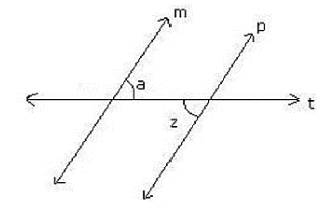19.

Calculate median and mode for following data:

$23,45,46,12,34,87,78,12,65,33,19,34,55,67,81,12,56,98,11,49,50$

20.

Find the value of the following expression using suitable property:

$725 times(-35)+(-725) times 65$

21.

Mala finished drinking a glass of milk in $frac{7}{8}$ minutes while varun took $frac{9}{16}$ minutes to drink the same amount of milk. Who took longer time and by how much?

22.

What will you get on subtracting $-134$ from the sum of 38 and $-87$ ?

23.

In a factory, $9.2$ kilograms of pumpkin pie filling is made per minute. How many kilograms of pie filling will be made in 6 minutes?

24.

Write the following equations in statement form:

i.

$6 n+4=10$

ii.

$frac{mathrm{y}}{7}-3=9$

Section C

(Questions 25 to 32 carry 3 marks each)

25.

Find the value of $mathrm{x}$ in the given figure.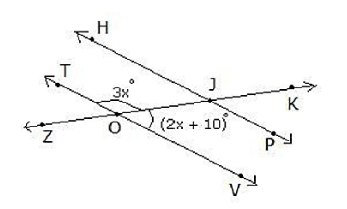26.

Simplify: $5.75-frac{3}{7} times 15 frac{3}{4}+2 frac{2}{35} div 1.44$

27.

The ages of Rahul and Karan are in the ratio $7: 5$. Ten years hence, the ratios of their ages will be $9: 7$. Find their present age?

28.

Find the values of $mathrm{x}$ and $mathrm{y}$ in the following figure.29.

In AABC shown below, $A D perp B C, B E perp A C$ and $A D=B E$. Prove that $A E=B D$.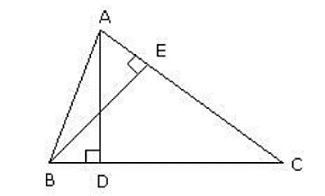30.

The bar graph given below shows the sales of books (in thousands) from six branches of a publishing company during two consecutive years 2000 and $2001 .$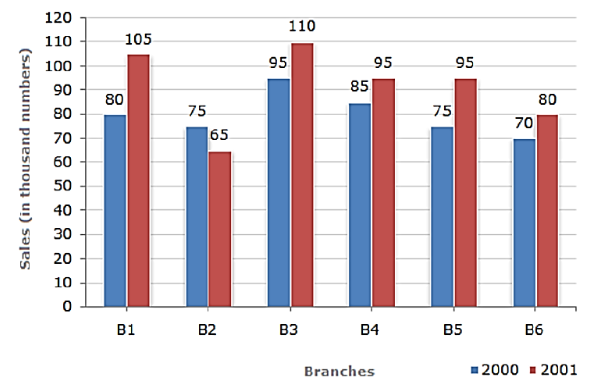i.

What is the ratio of the total sales of branch B2 for both years to the total ales of branch

B4 for both years?

ii.

What is the average sale of all the branches (in thousand numbers) for the year 2000 ?

iii.

Total sales of branch $mathrm{B} 6$ for both the years is what percent of the total sales of 4 branches B3 for both the years?

31.

f $frac{1}{2}$ of $frac{-3}{4}$ a number is 6, then what is the number?

32.

The given data is arranged in ascending order. The sum of mode and median of the given

data is 15 . Find the value of $mathrm{y}$.

$y-1, y-1, y+1, y+4,2 y+1,3 y, 4 y$

Section D

(Questions 33 to 37 carry 4 marks each)

33.

Given below are two triangles $mathrm{ABD}$ and $mathrm{CBD} . mathrm{AD}=mathrm{CD}$ and $angle 3=angle 4$. Prove that DB bisects

$angle mathrm{ABC} .$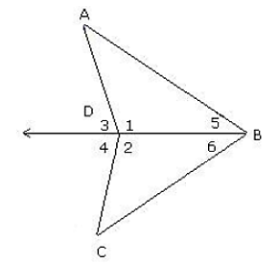34.

The number of trees planted by an agency in different years is given below:

 Years 1997 1998 1999 2000 2001 2002 Number of trees planted 400 450 700 750 900 1500

Draw a bar graph.

35.

Town A is 60 km from town B, and 61 km from town C. A road connects towns B and C
directly. Find the length of this road.

36.

Below are the numbers of goals scored in 1998 by the top five scorers on each of the three National Hockey League teams. Use this information to complete the chart.

 Team Goals Goals Goals Goals Goals Mean Median Mode India 35 25 30 19 19 Sri Lanka 45 25 14 13 14 China 32 18 14 21 21

37.

Simplify and reduce to standard form:

i.

$frac{-15}{35} timesleft(frac{27}{-63} div frac{81}{14}right)$

ii.

$left(frac{-2}{-72} div frac{4}{9}right) div frac{-6}{14}$

Privacy Settings